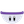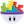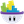#### Howdy, Stranger!

It looks like you're new here. If you want to get involved, click one of these buttons!

# How to make options that must include the correct answer

Posts: 10Memberedited March 2016

I would like to make a game which like a quiz (random question and random answers (including correct and wrong)), how can i make sure it can random a correct answer for player to choose in every round? Let's say,
2 + 2 = ?
1. 2
2. 3
3. 4
Make sure 4 must be appear. I was just used (random(1,10)).That mean, it must appear (5, 8, 9) or (5, 5, 3).
How can I fix the answer choices which must include 4 and not repeating show the neighbour numbers? if only using "if".."do", it is very long nest loop. Any other way to avoid?

• London, UK.Posts: 12,822Memberedited March 2016

Make a table with 10 rows, place the values 1-10 into each of the rows.
Make a Boolean attribute, let's call it 'Four'.

Select a random row for your first value.
If the value is 4 - then chage 'Four' to true.
Delete this row.

Select a random row for your second value.
If the value is 4 - then chage 'Four' to true.
Delete this row.

If 'Four' is true select a random row for your third value.
Otherwise make this value '4’

EDIT - just spotted an issue with this approach, the correct answer (in this case 4) will more often than not be the last answer, so you'do need to randomise these three selected answers (2 wrong, 1 correct), perhaps write them to a new three row table, and then randomly select (and delete / remove row) to randomise their order.

• Posts: 573Member, BASICAttached is a quick demo I made. It might be a little complicated.

• It picks 3 "wrong" answers from a table and 1 "Correct" Answer
• It then displays them in a random order (using Tables) so answer is not always in same spot.
• Touch each answer to see if correct (green) or wrong (red)
• Create new ones by pressing the bottom Actor.

Hope it helps

• Posts: 1,764MemberI made a little game like this and what I did was always have it add up the answer and then add or minus a random number from that to get a wrong choice worked well and no table needed.

• Posts: 4,771Member, PROHere's a slightly different method. Only uses the table that stores the correct and incorrect answers. This method assumes you want to pick 1 correct and 3 incorrect answers from a pool of 10. Easily enough adapted to other variations. If you want to randomise the incorrect answers entirely, that makes things way simpler.

• Posts: 10MemberThanks all, I used TWO.E's demo. But one more question, as my answer is a variable, it will be changed, so I cannot put "2016" as the actor attribute and the answer would put in the table, that means the answer would show repeated. Any way to filter the correct answer in the table?

• Posts: 573Member, BASIC@tammywkwong said:
Thanks all, I used TWO.E's demo. But one more question, as my answer is a variable, it will be changed, so I cannot put "2016" as the actor attribute and the answer would put in the table, that means the answer would show repeated. Any way to filter the correct answer in the table?

If I am understanding correctly, if you have a different answer for a different question, just change the "game.Answer" attribute to whatever the answer for that particular question. The demo puts the answer into the table of options, and 3 other wrong options from a table.

• Posts: 10MemberQuestion Solved! Thanks all !

This discussion has been closed.